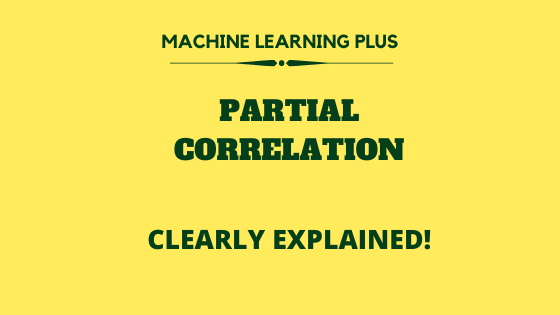Drop a Query

# Naveen James## Partial Correlation

What is Partial Correlation and it’s purpose Partial correlation is used to find the correlation between two variables (typically a dependent and an independent variable) with the effect of other influencing variables being controlled. For example, if there are three variables ‘A’, ‘B’, ‘Z’, If you want to find the relationship between ‘A’ and ‘B’ …## Chi-Square test – How to test statistical significance for categorical data?

What is chi-square test and its purpose? Chi-square test was invented in the year ‘1900’ by the revered mathematician ‘Karl Pearson’. Chi-square test, also written as χ2 test is used to determine whether there is a statistically significant difference between the observed frequency and the expected frequency in one or more categories of the contingency …Course Preview

## Machine Learning A-Z™: Hands-On Python & R In Data Science

### Free Sample Videos:#### Machine Learning A-Z™: Hands-On Python & R In Data Science#### Machine Learning A-Z™: Hands-On Python & R In Data Science#### Machine Learning A-Z™: Hands-On Python & R In Data Science#### Machine Learning A-Z™: Hands-On Python & R In Data Science